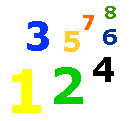# The Customary System

Measurement Lesson & Test - My Schoolhouse - Online Learning

Weights and measurements are used daily in our lives.  The United States Customary System uses the following units of measure for length, weight, and capacity.  The abbreviation for each unit is in parentheses.

 U.S. Customary System Length Weight Capacity inch (in) ounce (oz) cup (c) foot (ft) pound (lb) pint (pt) yard (yd) ton (t) quart (qt) mile (mi) gallon (gal)

Learn to change a measurement from one unit measure to another unit measure.  Look at the examples below.

 12 in = 1 ft 16 oz = 1 lb 2 c = 1 pt 3 ft = 1 yd 2000 lb = 1 t 2 pt = 1 qt 4 qt = 1 gal

Complete the following problems.

 12 in = ft 24 in = ft 36 in = ft3 ft = yd 1 yd = ft 36 in = yd2 yd = ft 6 ft = yd 72 in = yd16 oz = lb 32 oz = lb 4 lb = oz2 c = pt 2 pt = qt 4 qt = gal1 gal = c 3 qt = c 6 c = pt2 gal = pt 16 qt = gal 4 gal = c